Courses

# Simple Cost Sheet - Overheads B Com Notes | EduRev

## B Com : Simple Cost Sheet - Overheads B Com Notes | EduRev

The document Simple Cost Sheet - Overheads B Com Notes | EduRev is a part of the B Com Course Cost Accounting.
All you need of B Com at this link: B Com

Meaning of Cost Sheet:
Cost Sheet is a statement which presents detailed information relating to the various stages of cost. It also shows the total cost of the product manufactured during a particular period of time. Thus, the cost sheet is prepared for a particular period of time monthly, quarterly, yearly etc.

Objects of Preparing a Cost Sheet:
A cost sheet is prepared for:
(i) The total cost and cost per unit of the product can be ascertained;
(ii) It helps the management to fix up the selling price on the basis of the cost per unit of the product after charging certain percentage of profit on cost;
(iii) It also helps the management presenting a comparative study of current cost with the exis­ting cost per unit;
(iv) After proper comparison the management can take the corrective measures;
(v) It helps the management while formulating suitable production policy;
(vi) It is very helpful to submit a price quotation for tenders; and
(vii) It also helps the management by supplying suitable information for management control.

Method of Preparation of Cost Sheet:
Step I = Prime Cost = Direct Material + Direct Labour + Direct Expenses.
Step II = Works Cost = Prime Cost + Factory/Indirect Expenses.
Step III = Cost of Production = Works Cost + Office and Administration Expenses.
Step IV = Total Cost = Cost of Production + Selling and Distribution Expenses. Profit = Sales – Total Cost.
The above method can better be presented with the help of the following proforma Cost Sheet: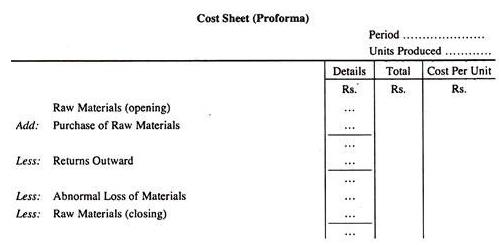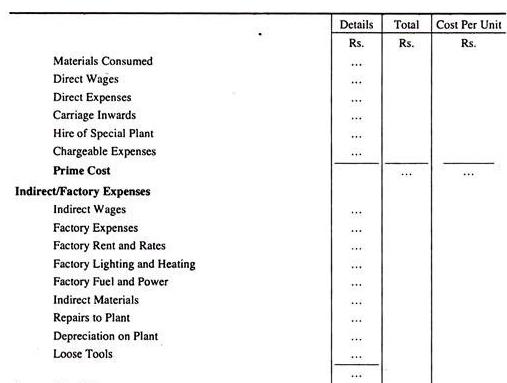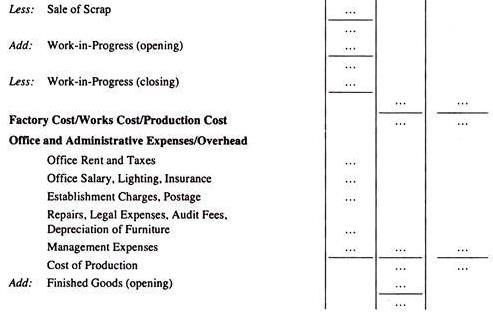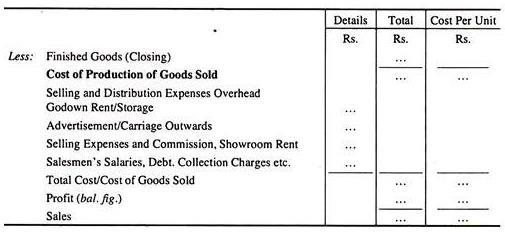Example and Solution.
Cost Sheet Problem 1:
From the following particulars of a manufacturing concern, ascertain the Prime Cost: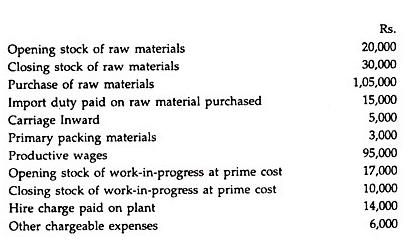Solution.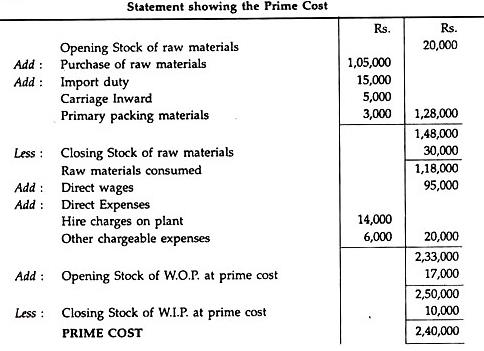Cost Sheet Problem 2:
From the following figures obtained from the costing records of product A ascertain the PRIME COST for the month of August 1997: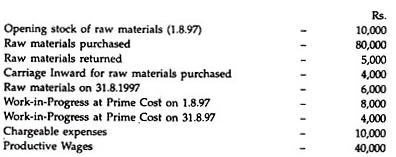Solution.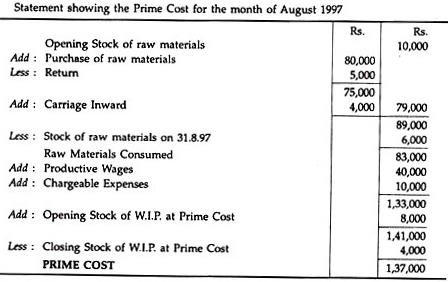Find out the Works Cost
Works Cost = Prime Cost + Factory Overheads + Opening Stock of Work-in-Progress at Factory Cost-Closing Stock of Work-in-Progress at Factory Cost.

Cost Sheet Problem 3:
From the following information ascertain the Works Cost for the month of August 1997: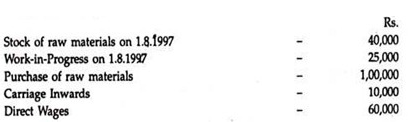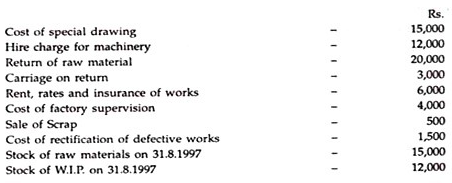Solution.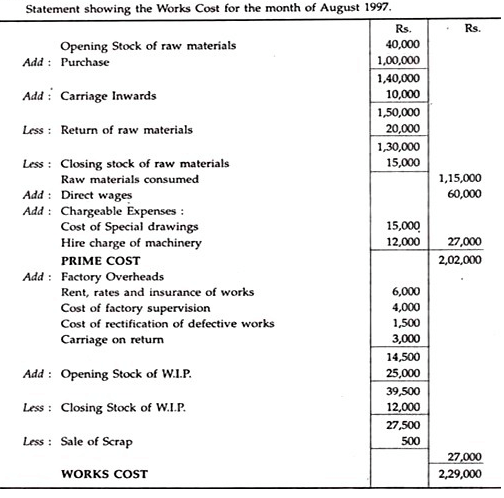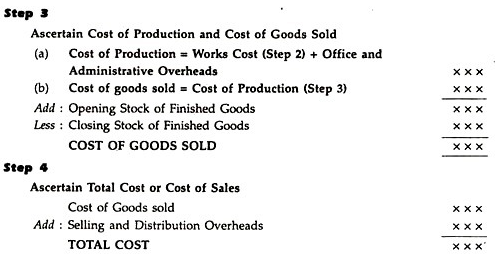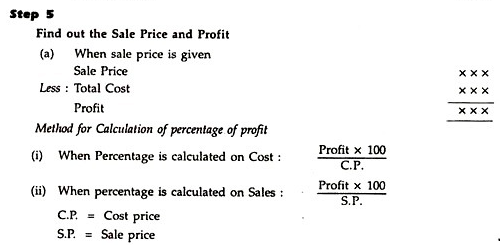Calculation of profit as a percentage of cost price or sales
(v) When profit is to be calculated as a percentage on sales but S.P. is not given.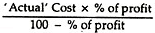Example: Assume that the cost of sales of a product A is Rs. 4,03,000. Now, the business wants to make profit at 20% on sales. We do not know the sale price. The amount of profit is to be calculted as follows :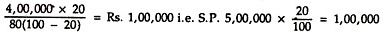Cost Sheet Problem 4:
The following particulars have been collected from a manufacturing concern for the year ended 31.3.1998: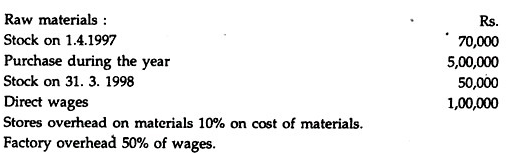10% on cost of materials. Factory overhead 50% of wages.
10% of the casting was rejected and a sum of 6,000 was realised on sale as scrap.
10% of finished products was found to be defective and the defective products were rectified at an additional expenditure which is equivalent to 20% of proportionate direct wages.
The total output was 10,000 tonnes during the year.
Ascertain the manufacturing cost per tonne.
Solution.
Statement of cost for the year ended 31st March 1998 – Output 9,000 tonnes.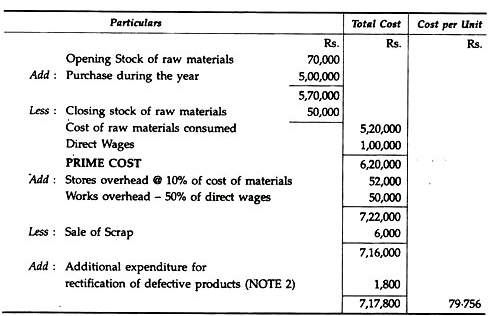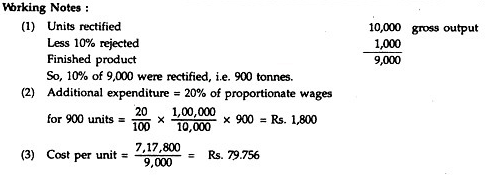Cost Sheet Problem 5:
The following particulars have been obtained from the cost records for the year 1997: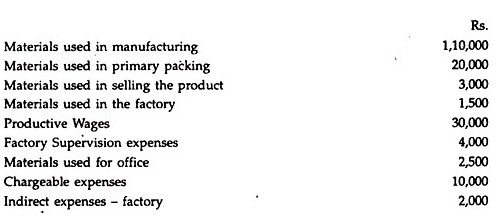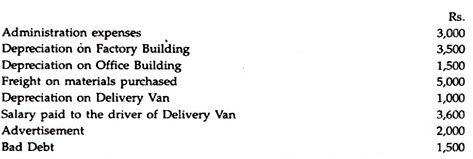Assume that all products manufactured during the year have been sold to earn a profit of 20% on selling price.
Solution.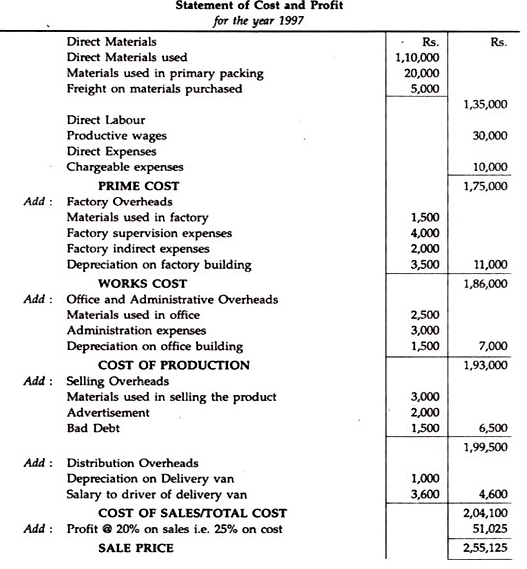Working Note : When sale price is 100, cost is Rs. 80 and Profit is Rs. 20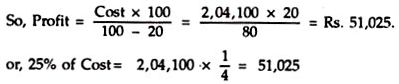Offer running on EduRev: Apply code STAYHOME200 to get INR 200 off on our premium plan EduRev Infinity!

## Cost Accounting

131 videos|139 docs

,

,

,

,

,

,

,

,

,

,

,

,

,

,

,

,

,

,

,

,

,

;